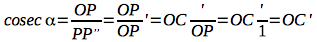#### 3.2 Graphs of trigonometric functions

###### Topography notes: Table of contentsWe consider a plane cartesian coordinate system, a circumference with radius r = 1 (this is most important!) and P point into that circumference.

Now we consider M =(0,1), the upper intersection between the circumference and Y-axis. Also we consider N=(1,0), the intersection on the right between the circumference and X-axis.

According to the definition of trigonometric functions we can write: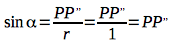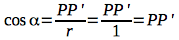In other words, the values of trigonometric functions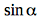and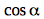are the length of the segments PP’ and PP”.

We are considering two straight lines: the line parallel to the x axis and passing through M, and the line parallel to the y axis. If we extend OP up to intersect these lines we obtain two points: T and C (see figure).

The triangles OPP” and OMT are similar, the triangles OPP’ and ONC are similar too. According to these similarities we can write: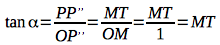and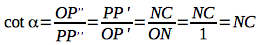Now if we consider the perpendicular line to OP passing through P, we obtain two intersect points: S and C’; respectively the intersection with y axis and x axis.

The triangles OPP” and OPS are similar, OPP’ and OPC’ are similar too. We can describe another two trigonometric functions: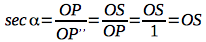and